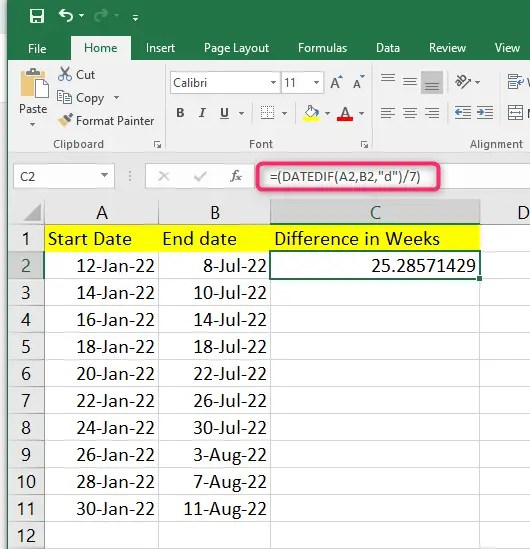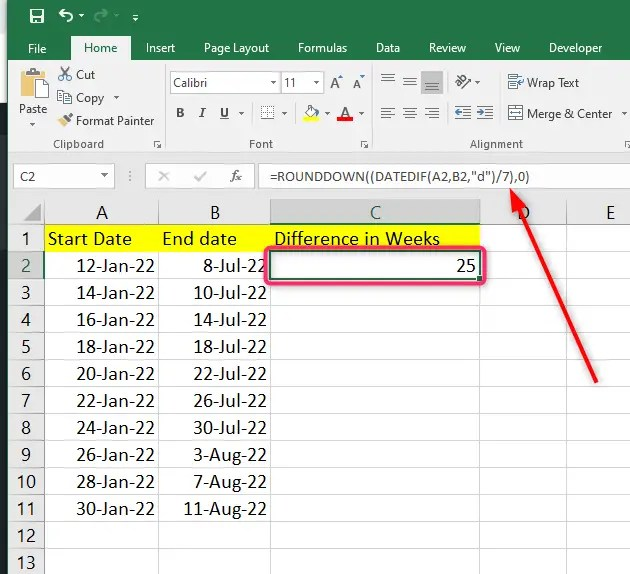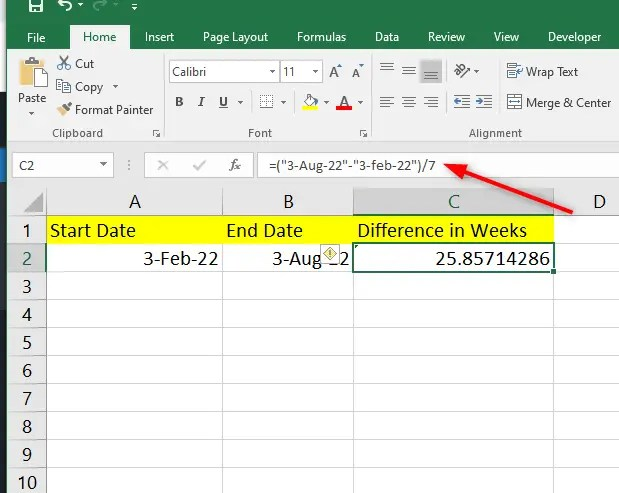# How to count weeks since date in Excel

Calculations and formulas are part of Excel. Any Excel user has to know how to work out the formulas faster for an efficient and effective workflow.  Also, knowing how to display week numbers in cells within your spreadsheets will help with reporting. Our guide below guides you on how to count weeks since the date in Excel.

## using the DATEDIF function

you can easily find the total number of weeks since the date. You can make use this function but add a ‘d’ unit into the formula to show the differences in days. Lastly, divide answer 7 to get  weeks.Use the formula:

=ROUNDDOWN ((DATEDIF (Start date, End date,“d”)/7),0)

1. Type this formula in an empty cell. Make sure you select cells representing your data’s start and end date.

2. Press Enter.a

3. Apply the formula to the remaining cells by dragging the autofill handle.Count weeks since date in Excel practice template

## Using the WEEKNUM function to count weeks since the date in Excel

You can also use the WEEKNUM function to count weeks in Excel. The function takes a date and returns a week number corresponding to the year’s week.

Here you will use the formula:

=WEEKNUM (“date”)

The formula above will give you the number of weeks (out of 54weeks) you are in within a year. In short, it will quickly determine how many weeks have elapsed since 1st January to a given date of the same year.The WEEKNUM formula only calculates the weeks from the specified date in that year.

## count number of weeks since date in Excel by subtracting

You can also count the number of weeks between two dates using

=(“End date” – “Start date”) / 7By subtracting the starting date from the date, you shall get the difference in days. Divide your results by 7 to get the total number of weeks since the date in Excel.

The formula above will result to a decimal. You can convert the decimal to a fraction to show the days. You can also decide to use the ROUND function to ROUND DOWN or UP to the closest whole number. To do this, you will click on Ctrl +1 to open the Format Cells dialog box.